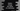# How to change the values of a dictionary in Python## How to change the values of a dictionary in Python:

In this post, we will learn how to change the values of a dictionary in Python. Python dictionaries are used to store key-value pairs. We can use the keys to access the value associated with that key. To change any value of the dictionary, we can use the key.

Let’s learn different ways to change the dictionary values in Python with examples.

### Example 1: Change the value of a Python dictionary by using its key:

Let’s learn how to change the value of a dictionary by using its key. We can refer an item with its key and change its value:

``````given_dict = {'one': 'Jan', 'two': 'Feb', 'three': 'March'}

print(f'Dictionary before change: {given_dict}')

given_dict['two'] = 2;

print(f'Dictionary after change: {given_dict}')``````

In this program, we changed the value of the dictionary with the key two. This program prints the value before and after the dictionary is updated.

It will print:

``````Dictionary before change: {'one': 'Jan', 'two': 'Feb', 'three': 'March'}
Dictionary after change: {'one': 'Jan', 'two': 2, 'three': 'March'}``````

As you can see here, it updated the value of the key ‘two’.

### Example 2: By using Python dictionary update() method:

The update method is an inbuilt method of python dictionary and it is used to update multiple key-value pairs of a dictionary at one go. It is defined as:

``dict.update(itr)``

This method takes one single parameter. It is an iterable with key-value pairs or another dictionary. It updates the key-value pairs of the original dictionary dict. It doesn’t return anything.

Let’s take a look at the below example:

``````given_dict = {'one': 'Jan', 'two': 'Feb', 'three': 'March'}
updated_dict = {'one': 1, 'two': 2}

print(f'Dictionary before change: {given_dict}')

given_dict.update(updated_dict)

print(f'Dictionary after change: {given_dict}')``````

In this example, we passed another dictionary updated_dict to the update() method which is called on given_dict. If you run this program, it will print:

``````Dictionary before change: {'one': 'Jan', 'two': 'Feb', 'three': 'March'}
Dictionary after change: {'one': 1, 'two': 2, 'three': 'March'}``````

If the keys are not found in the dictionary, it will append those pairs to the dictionary.

``````given_dict = {'one': 'Jan', 'two': 'Feb', 'three': 'March'}
updated_dict = {'four': 'April', 'five': 'May'}

print(f'Dictionary before change: {given_dict}')

given_dict.update(updated_dict)

print(f'Dictionary after change: {given_dict}')``````

In this example, the keys four and five are not in the original dictionary given_dict. So, these pairs will be appended to the dictionary.

``````Dictionary before change: {'one': 'Jan', 'two': 'Feb', 'three': 'March'}
Dictionary after change: {'one': 'Jan', 'two': 'Feb', 'three': 'March', 'four': 'April', 'five': 'May'}``````

### How to use Python dictionary update() method with an iterable:

We can also pass an iterable to the update() method instead of passing a dictionary.

``````given_dict = {'one': 'Jan', 'two': 'Feb', 'three': 'March'}
updated_dict = [("one", 1), ("two", 2), ("three", 3)]

print(f'Dictionary before change: {given_dict}')

given_dict.update(updated_dict)

print(f'Dictionary after change: {given_dict}')``````

We passed a tuple to the update method in this example. It will print similar result.

``````Dictionary before change: {'one': 'Jan', 'two': 'Feb', 'three': 'March'}
Dictionary after change: {'one': 1, 'two': 2, 'three': 3}``````